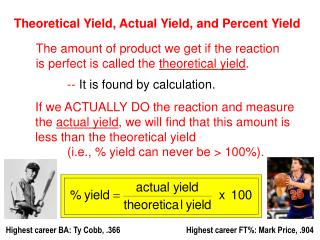DownloadDownload PresentationTheoretical Yield, Actual Yield, and Percent Yield

# Theoretical Yield, Actual Yield, and Percent Yield

Télécharger la présentation## Theoretical Yield, Actual Yield, and Percent Yield

- - - - - - - - - - - - - - - - - - - - - - - - - - - E N D - - - - - - - - - - - - - - - - - - - - - - - - - - -
##### Presentation Transcript

1. Theoretical Yield, Actual Yield, and Percent Yield The amount of product we get if the reaction is perfect is called the theoretical yield. -- It is found by calculation. If we ACTUALLY DO the reaction and measure the actual yield, we will find that this amount is less than the theoretical yield (i.e., % yield can never be > 100%). Highest career BA: Ty Cobb, .366 Highest career FT%: Mark Price, .904

2. ( ) . . _ _ . . ( ) ( ( ) ) ZnS+ O2ZnO+ SO2 2 3 2 2 100. g 100. g X g Assume 81% yield. Balance and find LR. Strategy: 1. 2. 3. Use LR to calc. X g ZnO (theo. yield) Actual yield is 81% of theo. yield. LR 1 mol = 0.513 100 g ZnS = 1.026 mol ZnS (HAVE) 2 97.5 g 1 mol 100 g O2 3 = 1.042 = 3.125 mol O2 (HAVE) 32 g ZnS ZnO 2 mol ZnO 81.4 g ZnO 1.026 mol ZnS = 83.5 g ZnO 1 mol ZnO 2 mol ZnS Actual g ZnO = 83.5 (0.81) = 68 g ZnO

3. 2 NaN3(s) 3 N2(g) + 2 Na(s) ( ( ) ) ( ) Automobile air bags inflate with nitrogen via the decomposition of sodium azide: At STP and a % yield of 85%, what mass sodium azide is needed to yield 74 L nitrogen? actual % yld = theo 74 L N2 theo = 87.1 L N2 0.85 = theo L N2 N2 NaN3 1 mol N2 2 mol NaN3 65 g NaN3 87.1 L N2 = 170 g NaN3 1 mol NaN3 3 mol N2 22.4 L N2